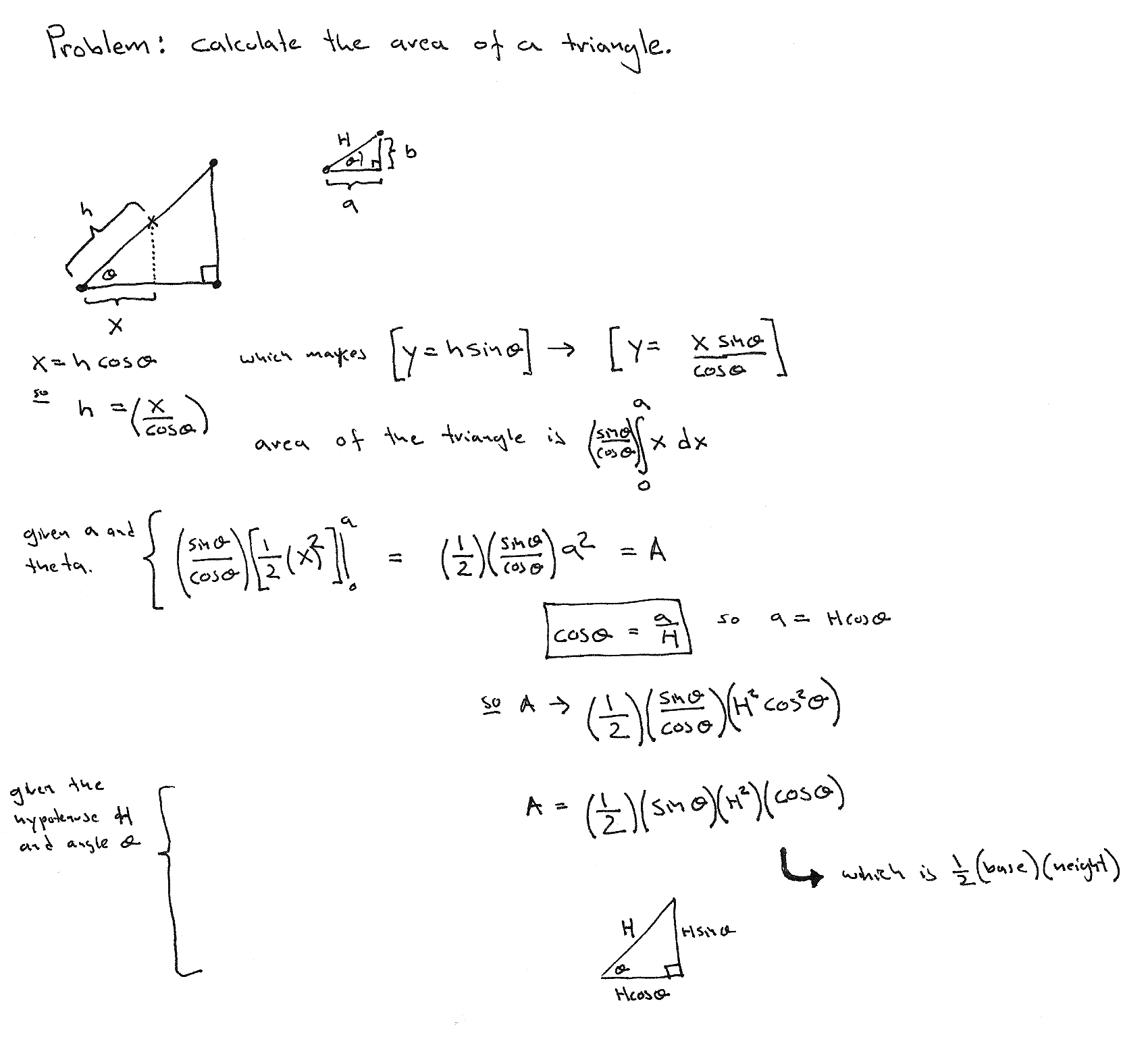# Find the rate problem

Problem 179 from http://mathproblems.info/group9.html

A drag racer accelerates at a uniform rate from its starting point. It travels the last one fourth of the distance from the starting point to the finish line in 3 seconds. How long did it take to travel the entire distance from starting point to finish line?

# Area of a right triangle

Ok, one of the first equations learned in school is that the area of a right triangle is half the base times the height. Obvious since the base times height gives the area of a rectangle and that is twice the are of the triangle in question. Using calculus though you can say the same thing as:Area of a right triangle via integration